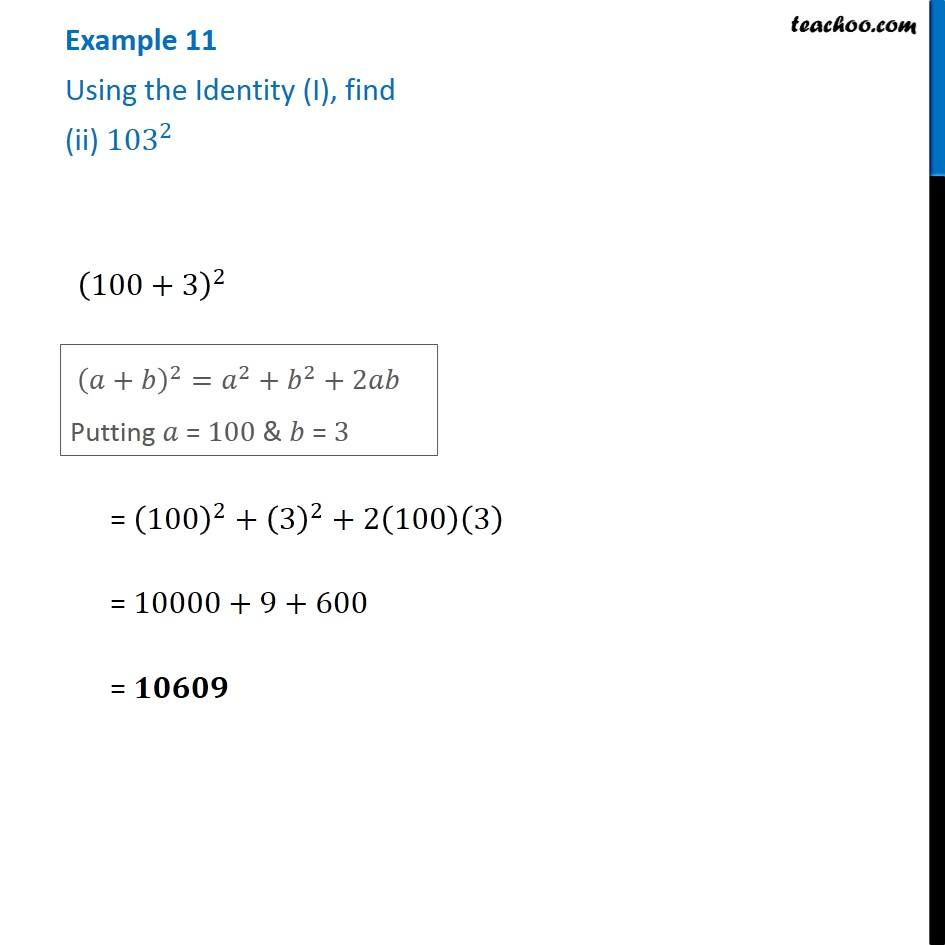Examples

Chapter 8 Class 8 Algebraic Expressions and Identities
Serial order wiseLearn in your speed, with individual attention - Teachoo Maths 1-on-1 Class

### Transcript

Example 11 Using the Identity (I), find (ii) 〖103〗^2 (100+3)^2 (𝑎+𝑏)^2=𝑎^2+𝑏^2+2𝑎𝑏 Putting 𝑎 = 100 & 𝑏 = 3 = (100)^2+(3)^2+2(100)(3) = 10000+9+600 = 𝟏𝟎𝟔𝟎𝟗